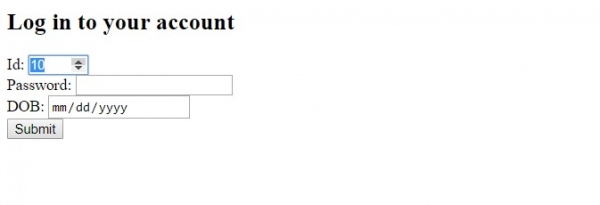# HTML <input> max Attribute

HTMLWeb DevelopmentFront End Technology

The max attribute of the <input> element is used to set the maximum value for <input>. Both min and max are used to set a range of value for input element with type number, date, datetime, range, etc. It introduced in HTML5.

Let us now see an example to implement the man attribute of the <input> element. Here, we have set max as 10, therefore a user cannot enter an ID more than 1 −

## Example

Live Demo

<!DOCTYPE html>
<html>
<body>
<form action="" method="get">
Id − <input type="number" name="id" min="1" max="10"><br>
DOB − <input type="date" name="dob" autofocus><br>
<button type="submit" value="Submit">Submit</button>
</form>
</body>
</html>

## OutputIn the above example, we have created a form with some fields and a button −

<form action="" method="get">
Id − <input type="number" name="id" min="1" max="10"><br>
</form>
Id − <input type="number" name="id" min="1" max="10">